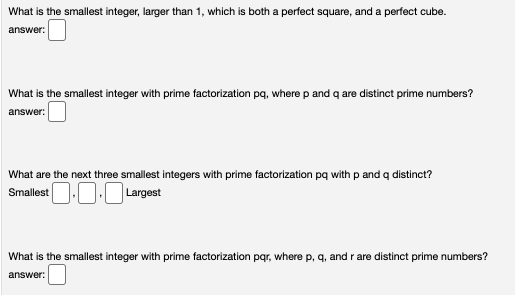# Question What is the smallest integer, larger than 1, which is both a perfect square, and a perfect cube. answer: What is the smallest integer with prime factorization pq, where p and q are distinct prime numbers? answer: What are the next three smallest integers with prime factorization pq with p and a distinct? Smallest ,0 Largest What is the smallest integer with prime factorization par, where p, q, and r are distinct prime numbers? answer:UX3NXN The Asker · Other MathematicsTranscribed Image Text: What is the smallest integer, larger than 1, which is both a perfect square, and a perfect cube. answer: What is the smallest integer with prime factorization pq, where p and q are distinct prime numbers? answer: What are the next three smallest integers with prime factorization pq with p and a distinct? Smallest ,0 Largest What is the smallest integer with prime factorization par, where p, q, and r are distinct prime numbers? answer:
More
Transcribed Image Text: What is the smallest integer, larger than 1, which is both a perfect square, and a perfect cube. answer: What is the smallest integer with prime factorization pq, where p and q are distinct prime numbers? answer: What are the next three smallest integers with prime factorization pq with p and a distinct? Smallest ,0 Largest What is the smallest integer with prime factorization par, where p, q, and r are distinct prime numbers? answer: